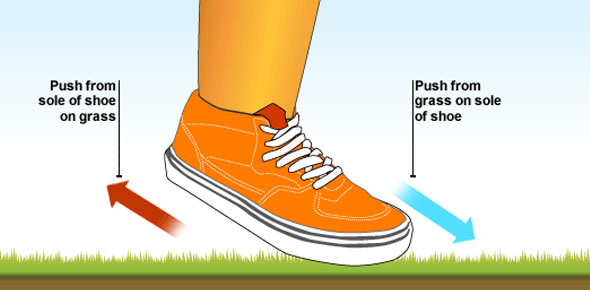# Matter In Motion Test

34 Questions | Attempts: 703SettingsQuiz to assess force and motion knowledge, as learned through the "Matter in Motion" lab.

• 1.
Friction is a force that
• A.

Opposes an object's motion.

• B.

Does not exist when surfaces are very smooth.

• C.

Decreases with larger mass.

• D.

All of the above.

• 2.
Rolling friction
• A.

Is usually less than sliding friction.

• B.

Makes it difficult to move objects on wheels.

• C.

Is usually greater than sliding friction.

• D.

Is the same as fluid friction.

• 3.
A force
• A.

Is expressed in newtons.

• B.

Can cause an object to speed up, slow down, or change direction.

• C.

Is a push or a pull.

• D.

All of the above.

• 4.
Which type of friction causes a sky diver's acceleration to change as she falls?
• A.

Sliding

• B.

Rolling

• C.

Fluid

• D.

Static

• 5.
Two forces act on an object.  One force has a magnitude of 10N and is directed toward the north.  The other has a magnitude of 5N directed toward the south.  The object experiences a net force of
• A.

5 N south

• B.

15 N north

• C.

50 N north

• D.

5 N north

• 6.
A reference point for determining position and motion could be
• A.

The Earth's surface.

• B.

A building.

• C.

A moving object.

• D.

All of the above.

• 7.
The distance traveled divided by the time it took to travel that distance determines an object's
• A.

Speed.

• B.

Acceleration.

• C.

Weight.

• D.

Force.

• 8.
You are in Chicago, IL.  You decide to head south to Austin, TX.  In one hour, you travel 80 km.  Your velocity is
• A.

80:1

• B.

80 km

• C.

80 km/h

• D.

80 km/h south

• 9.
A car traveling 20 m/s south enters a new highway going east at 20 m/s.  The car has
• A.

Decreased its distance.

• B.

Changed its speed.

• C.

Accelerated.

• D.

Not changed its velocity.

• 10.
Acceleration is a change in
• A.

Speed.

• B.

Velocity.

• C.

Direction.

• D.

All of the above.

• 11.
When velocity decreases, this could be referred to as
• A.

Acceleration.

• B.

Deceleration.

• C.

Negative acceleration.

• D.

All of the above.

• 12.
Which of the following is NOT an example of a force being exerted?
• A.

Pushing a door open

• B.

Typing on a computer keyboard

• C.

Sitting in a chair

• D.

None of the above

• 13.
A friend is helping you to arrange your bedroom furniture.  You exert 25 N of force to push the foot-end of the bed while your friend exerts 20 N of force pulling on the head-end of the bed.  The net force being used to move the bed is
• A.

5 N forward

• B.

20 N forward

• C.

25 N forward

• D.

45 N forward

• 14.
What is the net force when you combine a force of 7 N north with a force of 5 N south?
• A.

2 N north

• B.

2 N south

• C.

12 N north

• D.

12 N south

• 15.
Balanced forces applied to an object
• A.

Produce a net force of zero.

• B.

Change the direction of a moving object.

• C.

Cause an object at rest to start moving.

• D.

Produce negative net force.

• 16.
A good rule to observe when around a swimming pool is "no running."  The deck around the pool becomes very slippery to walk on when wet due to
• A.

Sliding friction.

• B.

Rolling friction.

• C.

Fluid friction.

• D.

Static friction.

• 17.
One way to increase friction is to use
• A.

Wax.

• B.

Water.

• C.

Sand.

• D.

Oil.

• 18.
The air that comes out of the tiny holes of an air-hockey table is an example of
• A.

Net force.

• B.

Friction.

• C.

Gas lubricant.

• D.

Liquid lubricant.

• 19.
An object's velocity remain constant when its __________ do not change.
• A.

Speed and direction

• B.

Speed and time in motion

• C.

Time in motion and direction

• D.

Distance traveled and time in motion

• 20.
Your class and another class have a tug-of-war contest.  For your class to win, your class must
• A.

Create a balanced force with the other class.

• B.

Have a greater force in the direction opposite the other class.

• C.

Have a greater force in the same direction as the other class.

• D.

Create a net force of zero with the other class.

• 21.
You have made a house of cards on top of your table.  Suddenly, a gust of wind blows through an open window and your house of cards comes tumbling down.  The wind applied ______ to your house of cards.
• A.

Friction

• B.

A balanced force

• C.

An unbalanced force

• D.

A gravitational force

• 22.
When applying brakes on a car or a bicycle, you are using _______ friction to stop.
• A.

Sliding

• B.

Rolling

• C.

Fluid

• D.

Static

• 23.
_______________ opposes motion between surfaces that are touching. (Friction or Gravity)
• 24.
Forces are expressed in _______________. (newtons or mass)
• 25.
A _______________ is determined by combining forces. (net force or newton)

## Related TopicsBack to top
×

Wait!
Here's an interesting quiz for you.